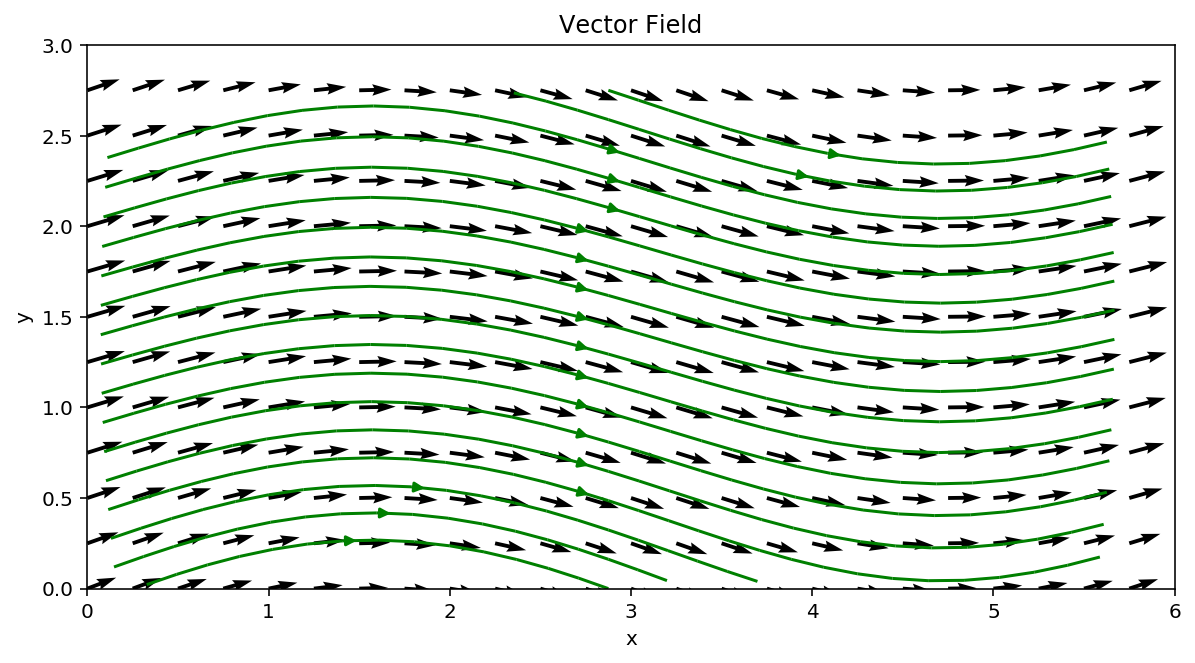# Derivation of Bernoulli's Equations¶

## Learning outcomes¶

• Learn how Bernoulli's equations are derived.
• Work in curvilinear coordinates
• Learn about some applications of Bernoulli's equations.

## Recap of Vector fields¶

As we saw in the previous notebook, the velocity of a fluid flow at each point in an Eulerian sense can be represented by a vector field $\vec{V}(\vec{x},t)$ where the position vector $\vec{x}$ denotes the position of each velocity vector relative to the origin, $\vec{x}(x,y,z) = x\mathbf{\hat{i}}+y\mathbf{\hat{j}}+z\mathbf{\hat{k}}$ where $\mathbf{\hat{i}}$, $\mathbf{\hat{j}}$ and $\mathbf{\hat{k}}$ are unit basis vectors, and the current time is given by $t$. Any position vector can be described extending from the origin to some point $(x,y,z)$. Similarly $\vec{V}({\vec{x}},t) = u\mathbf{\hat{i}}+v\mathbf{\hat{j}}+w\mathbf{\hat{k}}$ where $u={u({\vec{x}}},t)$, $v={v({\vec{x}}},t)$ and $w={w({\vec{x}}},t)$ are the velocity magnitudes in each Cartesian direction for the current time, $t$. An example 2D flow field is shown below. Each vector's tail is positioned on the Cartesian grid according to its position vector $\vec{{}x}$ and the velocity vector field, which in this case is time invariant, is given by:

\begin{equation} \vec{{}V}(x,y) = (\sin(y)+\pi) \mathbf{\hat{{}i}} + 1.2\cos(x) \mathbf{\hat{{}j}}, \end{equation}

which results in a flow from left to right with a slight sinuous meander in the $y$ direction. We can plot this and also include some streamlines.A simple 2D vector field created in MATLAB; can you recreate it?

Can you recreate this flow field with MATLAB or Python?

In :
import numpy as np
import matplotlib.pyplot as plt

%matplotlib inline
%config InlineBackend.figure_format = 'retina'

# Generate position vectors
x = np.arange(0, 6, 0.25)
y = np.arange(0, 3, 0.25)

X, Y = np.meshgrid(x, y)

# Velocity components
u = np.sin(Y) + np.pi
v = 1.2*np.cos(X)

# Create a new figure axes
fig, ax = plt.subplots(figsize=(10,5))

# Plot the vector field
ax.quiver(X, Y, u, v)

# Number of streamlines we want to draw
nstream = 18

# Points in (x,y) where the streamlines start (they are then integrated in both directions)
# In this case I take nstream points along from the lowest y value to the highest along
# the mid point of x. Rather than type actual numbers this method allows the code to work
# with any input should you change x and y
seed_points = np.array([np.linspace(np.median(x), np.median(x),nstream),\
np.linspace(np.min(y), np.max(y),nstream)])

# plot the streamlines
ax.streamplot(X, Y, u, v,color='g',start_points=seed_points.T)

plt.xlabel("x")
plt.ylabel("y")
plt.title("Vector Field")
plt.xlim([0, 6])
plt.ylim([0, 3])
ax.set_aspect('equal')
plt.show()Streamlines, as we have already seen, are simply curves that are everywhere tangent to the vector field for any instance in time. This means that the flow is everywhere following the curvature of the streamline and hence never crosses them. There is no mass flux normal to a streamline. The sinusoidal nature of the flow field shown above is now readily apparent by employing streamlines to visualise the flow.

## Streamline orientated coordinate system¶

Let us now consider a new coordinate system, $(s,n)$, mapped to the curvature of the streamlines. The position of any particle and its velocity in a steady flow can described in terms of its position along a streamline $s$ and its position normal to that streamline $n$. Unlike our simple Cartesian coordinate system this new $(s,n)$ system can be curvilinear but don't worry, it's much the same.

The figure below shows the green streamlines from previous figure as well as the curves normal to them, shown in red. As above the flow is still confined to the streamlines with no velocity normal along the red lines. Notice how as the green streamlines curve upwards the red normal curves fan outwards.The s-n coordinate system for the velocity field defined above

Lets try to recreate this image with Python and matplotlib.

In :
# recreate our position vector grid
x = np.arange(0, 6, 0.2)
y = np.arange(0, 3, 0.2)

X, Y = np.meshgrid(x, y)

# components of velocity field
u = np.sin(Y) + np.pi
v = 1.5*np.cos(X)

# Create a new figure axes
fig, ax = plt.subplots(figsize=(16,8))

# Plot the vector field
ax.quiver(X, Y, u, v)

# We can make this easy by plotting our green streamlines and
# then plotting red streamlines by flipping the velocity components
# This is a simple inplementation of a 'rotation matrix'

# Becasue we want to define starting points for both sets of streamlines
# we can define a function that we can call upon as needed
# Its good practise to avoid repeating code

def gen_seeds(x,y,nstream):
seed_points = np.array([np.linspace(np.median(x), np.median(x),nstream),\
np.linspace(np.min(y), np.max(y),nstream)])
return seed_points

# As above we want to seed our streamlines evenly from top to bottom from the midpoint in x
# our function gen_seeds is designed to do this
seed_points = gen_seeds(x,y,20)

ax.plot(seed_points,seed_points,'go')
ax.streamplot(X, Y, u, v,color='green',start_points=seed_points.T)

# For the red streamlines we need to seed from left to right about the midpoint in y
# so we feed it (y,x) instead of (x,y) and then flip the result back to (x,y)
seed_points = np.flipud(gen_seeds(y,x,20))

ax.plot(seed_points,seed_points,'ro')
ax.streamplot(X, Y, v, -u,color='red',start_points=seed_points.T)

plt.xlabel("x")
plt.ylabel("y")
plt.title("$(s,n)$ Coordinate System")
plt.xlim([0, 6])
plt.ylim([0, 3])
ax.set_aspect('equal')
plt.show()